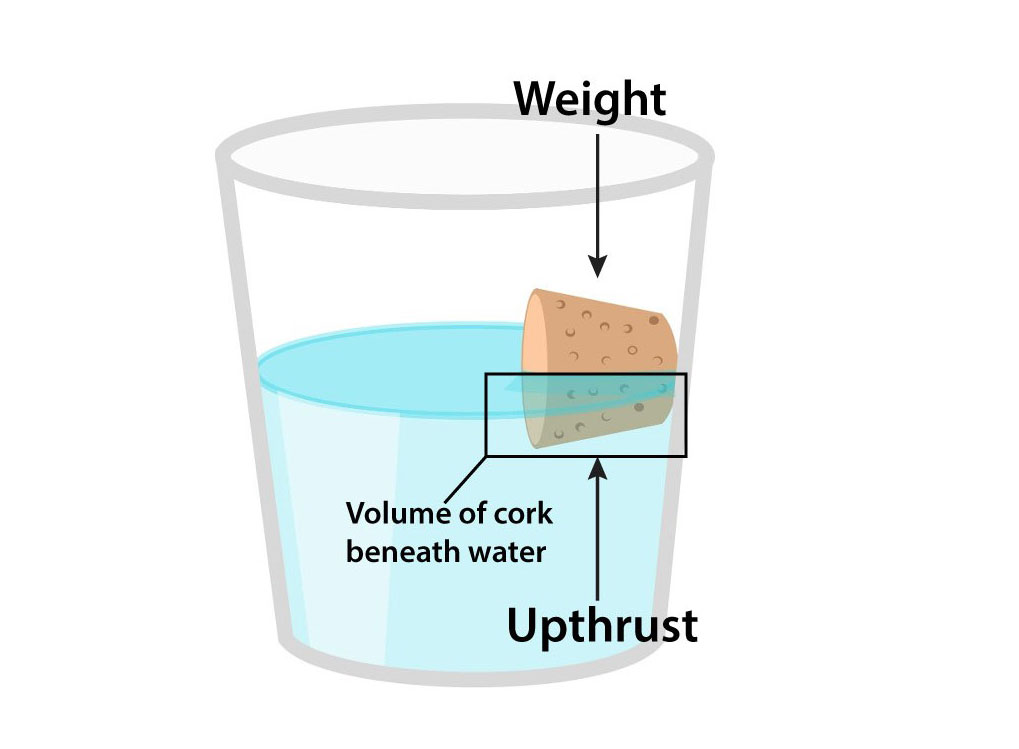• SECTION 01

(a)(i) What peculiar property does the effort has in all classes of levers?

Force creates efforts for different types of levers
-In class 1 of levers effort situated at the end after pivot at the middle
-In class 2 of levers effort situated at the end after load at the middle
-In class 3 of levers effort situated at the middle of load and pivot(ii) A meter rule of weight 1.0 N is supported horizontally on two knife edges each placed 10.0 cm from its ends. If the weight of 1.5 N is placed at its mid-point, calculate the reaction at the supports.

Data and Reasoning:

Weight of the ruler = 1N (assumed to be located at the center of ruler)

Force located at the center = 1.5N

Distance near the edges = 0.1m

At equilibrium, sum of clockwise moments = sum of counter clockwise moment, hence 0.4R1 = 0.4R2 … (i)

At equilibrium, sum of upward forces = sum of downward forces, hence R1 + R2 = 2.5 … (ii)

Solving two equations, R1 = 1.25N and R2 = 1.25N

• SECTION 02

(b)(i) State the law of floatation.

"Floating object displace its own weight on fluid it floats."

(ii) Find the fraction of the cork that partially immersed when a piece of cork of density 0.25 g/cm 3 and a mass of 20 g floats in water.Data and solution:

Fraction of cork immersed corresponds to volume of liquid displaced

Volume displaced corresponds to up-thrust from water

For floating objects, up-thrust, U = weight of an object, W (application of Law of floatation)

So, Up-thrust (U) = Mass x gravity = 0.020Kg x10N/Kg = 0.2 N

Also, Up-thrust (U) = Weight of fluid displaced (Wd) = 0.2N of water (application of Archimedes principle)

- What is volume of water with weight of 0.2N?

- Weight = Mass x acceleration due to gravity and Mass = Density x Volume

- Weight = Density x Volume x acceleration due to gravity = ρvg

- V = Weight / (Density x Acceleration due to gravity) = 0.2N/(1000Kg/mx 10N/Kg) = 0.00002m= 20cm3 of water

- Since volume of water displaced is equal volume of cork under water, so volume of cork under water = 20 cm

But the question ask for fraction, so what is the total volume?

- Volume = m/ρ = 20g / 0.25gcm3 = 80cm3

- So fraction = 20cm3/ 80cm3 = ¼  Ans.